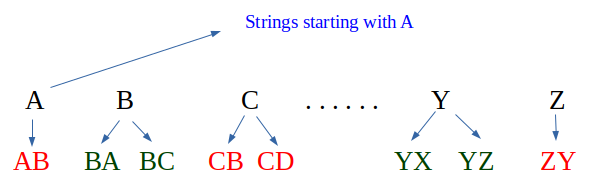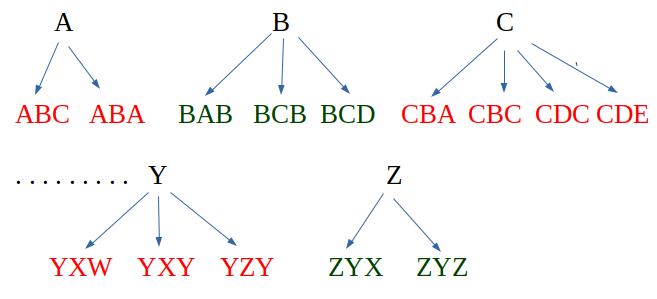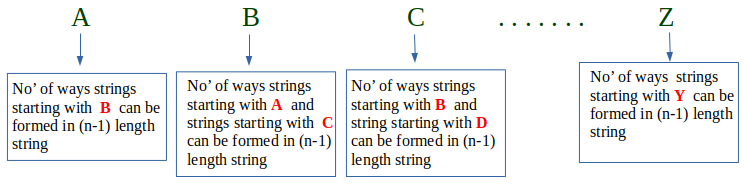GFG App
Open AppBrowser
Continue

# Count of strings where adjacent characters are of difference one

Given a number n, count the number of strings of length n such that every string has adjacent characters with a difference between ASCII values as 1.

Examples

```Input :  N = 1
Output : Total strings are 26
Explanation : For N=1, strings
are a, b, c,, ...., x, y, z

Input :  N = 2
Output : Total strings are 50
Explanation : For N = 2, strings
are ab, ba, bc, cb, .., yx, yz, zy```

For strings starting with character ‘A’ and length ‘i’, we consider all strings of length ‘i-1’ and starting with character ‘B’
For strings starting with character ‘G’ and length ‘i’, we consider all strings of length ‘i-1’ and starting with character ‘H’ and all strings of length ‘i-1’ and starting with ‘F’.
We take the base case for n = 1, and set result for all 26 characters as 1. This simply means when 1 character string is consider all alphabets from a-z are taken only once.
For N = 2For N = 3Conclusion : For N = n```countAdjacent(n)
dp[i][j] finally stores count of strings
of length i and starting with
character j.

Initialize dp[n+1] as 0
Initialize dp[j] = 1 where j = 0 to 25
for i = 2 to n
for j = 0 to 25
if (j = 0)
dp[i][j] = dp[i-1][j+1];
else
dp[i][j] = dp[i-1][j-1] + dp[i-1][j+1];
Sum of n-th row from 0 to 25 is the result.```

Implementation:

## C++

 `// CPP Program to count strings with adjacent` `// characters.` `#include ` `using` `namespace` `std;`   `int` `countStrs(``int` `n)` `{` `    ``long` `int` `dp[n + 1];`   `    ``// Initializing arr[n+1] to 0` `    ``memset``(dp, 0, ``sizeof``(dp));`   `    ``// Initializing 1st row all 1 from 0 to 25` `    ``for` `(``int` `i = 0; i <= 25; i++)` `        ``dp[i] = 1;`   `    ``// Begin evaluating from i=2 since 1st row is set` `    ``for` `(``int` `i = 2; i <= n; i++) {` `        ``for` `(``int` `j = 0; j <= 25; j++)`   `            ``// j=0 is 'A' which can make strings ` `            ``// of length i using strings of length ` `            ``// i-1 and starting with 'B'` `            ``if` `(j == 0) ` `                ``dp[i][j] = dp[i - 1][j + 1];` `            ``else` `                ``dp[i][j] = (dp[i - 1][j - 1] +` `                            ``dp[i - 1][j + 1]);` `    ``}`   `    ``// Our result is sum of last row.` `    ``long` `int` `sum = 0;` `    ``for` `(``int` `i = 0; i <= 25; i++)` `        ``sum = (sum + dp[n][i]);` `    ``return` `sum;` `}`   `// Driver's Code` `int` `main()` `{` `    ``int` `n = 3;` `    ``cout << ``"Total strings are : "` `<< countStrs(n);` `    ``return` `0;` `}`

## Java

 `// Java Program to count strings` `// with adjacent characters.` `import` `java.io.*;` `class` `GFG {`   `    ``static` `long` `countStrs(``int` `n)` `    ``{` `        ``long``[][] dp = ``new` `long``[n + ``1``][``27``];`   `        ``// Initializing arr[n+1] to 0` `        ``for` `(``int` `i = ``0``; i < n + ``1``; i++) {` `            ``for` `(``int` `j = ``0``; j < ``27``; j++) {` `                ``dp[i][j] = ``0``;` `            ``}` `        ``}`   `        ``// Initializing 1st row all 1 from 0 to 25` `        ``for` `(``int` `i = ``0``; i <= ``25``; i++) {` `            ``dp[``1``][i] = ``1``;` `        ``}`   `        ``// Begin evaluating from i=2` `        ``// since 1st row is set` `        ``for` `(``int` `i = ``2``; i <= n; i++) {`   `            ``// j=0 is 'A' which can make strings` `            ``for` `(``int` `j = ``0``; j <= ``25``; j++)`   `            ``// of length i using strings of length` `            ``// i-1 and starting with 'B'` `            ``{` `                ``if` `(j == ``0``) {` `                    ``dp[i][j] = dp[i - ``1``][j + ``1``];` `                ``}` `                ``else` `{` `                    ``dp[i][j] = (dp[i - ``1``][j - ``1``]` `                                ``+ dp[i - ``1``][j + ``1``]);` `                ``}` `            ``}` `        ``}`   `        ``// Our result is sum of last row.` `        ``long` `sum = ``0``;` `        ``for` `(``int` `i = ``0``; i <= ``25``; i++) {` `            ``sum = (sum + dp[n][i]);` `        ``}` `        ``return` `sum;` `    ``}`   `    ``// Driver Code` `    ``public` `static` `void` `main(String[] args)` `    ``{` `        ``int` `n = ``3``;` `        ``System.out.println(``"Total strings are : "` `                           ``+ countStrs(n));` `    ``}` `}`   `// This code is contributed by 29AjayKumar`

## Python 3

 `# Python3 Program to count strings with` `# adjacent characters. ` `def` `countStrs(n):`   `    ``# Initializing arr[n+1] to 0 ` `    ``dp ``=` `[[``0` `for` `j ``in` `range``(``27``)] ` `             ``for` `i ``in` `range``(n ``+` `1``)]`   `    ``# Initializing 1st row all 1 from 0 to 25` `    ``for` `i ``in` `range``(``0``, ``26``):` `        ``dp[``1``][i] ``=` `1`   `    ``# Begin evaluating from i=2 since ` `    ``# 1st row is set     ` `    ``for` `i ``in` `range``(``2``, n ``+` `1``):` `        ``for` `j ``in` `range``(``0``, ``26``):`   `            ``# j=0 is 'A' which can make strings ` `            ``# of length i using strings of length ` `            ``# i-1 and starting with 'B' ` `            ``if``(j ``=``=` `0``):` `                ``dp[i][j] ``=` `dp[i ``-` `1``][j ``+` `1``];` `            ``else``:` `                ``dp[i][j] ``=` `(dp[i ``-` `1``][j ``-` `1``] ``+` `                            ``dp[i ``-` `1``][j ``+` `1``])`   `    ``# Our result is sum of last row.         ` `    ``sum` `=` `0` `    ``for` `i ``in` `range``(``0``, ``26``):` `        ``sum` `=` `sum` `+` `dp[n][i]`   `    ``return` `sum` `    `  `# Driver's Code ` `if` `__name__ ``=``=` `"__main__"``:` `    ``n ``=` `3` `    ``print``(``"Total strings are : "``, countStrs(n))` `    `  `# This code is contributed by Sairahul Jella`

## C#

 `// C# Program to count strings with  ` `// adjacent characters. ` `using` `System; `   `class` `GFG ` `{ ` `    ``static` `long` `countStrs(``int` `n) ` `    ``{ ` `        ``long``[,] dp = ``new` `long``[n + 1, 27]; ` `    `  `        ``// Initializing arr[n+1] to 0 ` `        ``for``(``int` `i = 0; i < n + 1; i++)` `            ``for``(``int` `j = 0; j < 27; j++)` `                ``dp[i, j] = 0;` `    `  `        ``// Initializing 1st row all 1 from 0 to 25 ` `        ``for` `(``int` `i = 0; i <= 25; i++) ` `            ``dp[1, i] = 1; ` `    `  `        ``// Begin evaluating from i=2 since 1st row is set ` `        ``for` `(``int` `i = 2; i <= n; i++)` `        ``{ ` `            ``for` `(``int` `j = 0; j <= 25; j++) ` `    `  `                ``// j=0 is 'A' which can make strings ` `                ``// of length i using strings of length ` `                ``// i-1 and starting with 'B' ` `                ``if` `(j == 0) ` `                    ``dp[i, j] = dp[i - 1, j + 1]; ` `                ``else` `                    ``dp[i, j] = (dp[i - 1, j - 1] + ` `                                ``dp[i - 1, j + 1]); ` `        ``} ` `    `  `        ``// Our result is sum of last row. ` `        ``long` `sum = 0; ` `        ``for` `(``int` `i = 0; i <= 25; i++) ` `            ``sum = (sum + dp[n, i]); ` `        ``return` `sum; ` `    ``} ` `    `  `    ``// Driver Code` `    ``static` `void` `Main() ` `    ``{ ` `        ``int` `n = 3; ` `        ``Console.Write(``"Total strings are : "` `+ countStrs(n)); ` `    ``}` `}`   `// This code is contributed by DrRoot_`

## Javascript

 ``

Output

`Total strings are : 98`

Time Complexity: O(26*n)
Auxiliary Space: O(26*n)

This article is contributed by Shubham Rana. If you like GeeksforGeeks and would like to contribute, you can also write an article using write.geeksforgeeks.org or mail your article to review-team@geeksforgeeks.org. See your article appearing on the GeeksforGeeks main page and help other Geeks.

My Personal Notes arrow_drop_up